Question

5.) How many milliliters of a 1.50 molar copper (II) sulfate solution contain 25.0 grams of...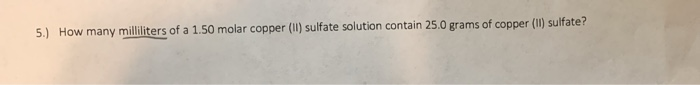5.) How many milliliters of a 1.50 molar copper (II) sulfate solution contain 25.0 grams of copper (II) sulfate?

molarity = (W/MW)(1000/V in mL)

molarity = 1.50 M

W = 25.0 g

MW = 159.61 g/mol

V = ?

1.50 = (25.0 / 159.61)(1000 / V)

1000/V = 9.58

V = 104.4 mL

Earn Coins

Coins can be redeemed for fabulous gifts.

Similar Homework Help Questions
• A solution was found to contain 5.21% copper (II) sulfate. How many grams of solution would...

A solution was found to contain 5.21% copper (II) sulfate. How many grams of solution would contain 25.0 g of copper (II) sulfate?

• 5. How many milliliters of a 20% magnesium sulfate solution will contain 40 grams of the...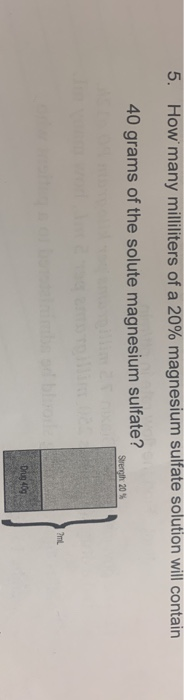5. How many milliliters of a 20% magnesium sulfate solution will contain 40 grams of the solute magnesium sulfate? Strength 20% Drug 409

• How many milliliters of an aqueous solution of 0.182 M copper(II) sulfate is needed to obtain...

How many milliliters of an aqueous solution of 0.182 M copper(II) sulfate is needed to obtain 10.3 grams of the salt?

• 3. How many milliliters of a 20% magnesium sulfate solution will contain 40 grams of the...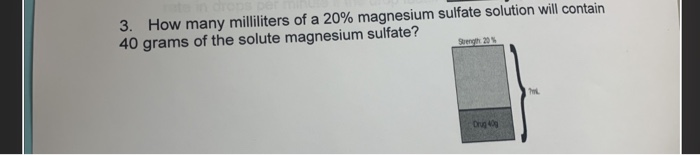3. How many milliliters of a 20% magnesium sulfate solution will contain 40 grams of the solute magnesium sulfate?

• how many milliliters of 20% HBr solution contain 4 grams of HBr? 2. How many milliliters...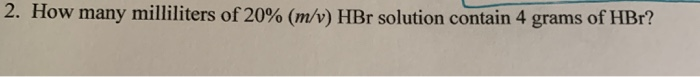how many milliliters of 20% HBr solution contain 4 grams of HBr? 2. How many milliliters of 20% (m/v) HBr solution contain 4 grams of HBr?

• how many grams of copper(II) sulfate would be needed to prepare 100.00mL of a 3.50 M...

how many grams of copper(II) sulfate would be needed to prepare 100.00mL of a 3.50 M solution?

• Determine the mass (in grams) of a 1% solution of Manganese(II) Sulfate that will contain grams...

Determine the mass (in grams) of a 1% solution of Manganese(II) Sulfate that will contain grams of Manganese(II) Sulfate.

• hector is performing a chemistry experiment that requires 140 milliliters of a 30% copper sulfate solution

hector is performing a chemistry experiment that requires 140 milliliters of a 30% copper sulfate solution. He has a 25% copper sulfate solution and a 60% copper sulfate solution. How many milliliters of each solution should he mix to obtain the needed solution?

• 5. How many milliliters (mL) of a 3.277 M calcium nitrate solution would contain 76.50 grams...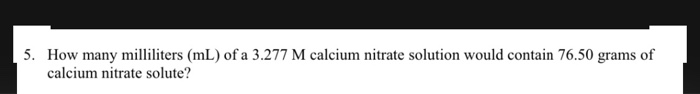5. How many milliliters (mL) of a 3.277 M calcium nitrate solution would contain 76.50 grams of calcium nitrate solute?

• How many grams of nickle (II) sulfate NiSO4(molar mass of 154.8g/mol) must be dissolved in 288.0g...

How many grams of nickle (II) sulfate NiSO4(molar mass of 154.8g/mol) must be dissolved in 288.0g of water to raise the boiling point by 0.350 C Kbp 0.51 Please Provide step by step solution